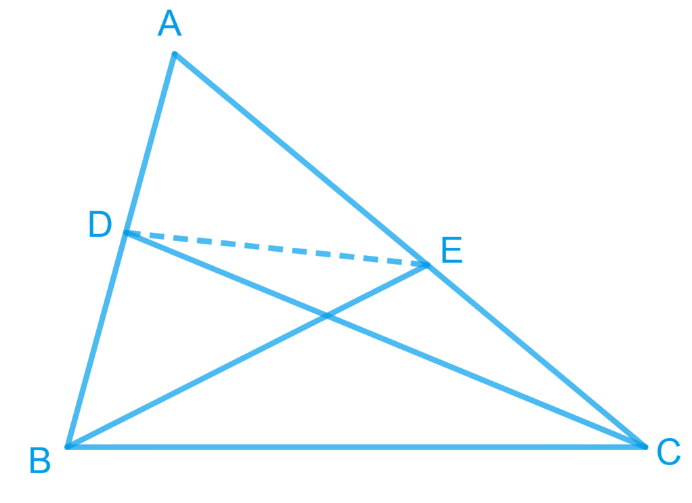# Ex.9.3 Q7 Areas of Parallelograms and Triangles Solution - NCERT Maths Class 9

## Question

$$D$$ and $$E$$ are points on sides $$AB$$ and $$AC$$ respectively of $$\Delta ABC$$ such that $$\text{ar }(DBC) = \text{ar }(EBC)$$. Prove that $$DE\; \| \;BC$$.

Video Solution
Areas Of Parallelograms And Triangles
Ex 9.3 | Question 7

## Text Solution

What is known?

$$D$$ and $$E$$ are points on sides $$AB$$ and $$AC$$ respectively of such that $$ar (DBC) = ar (EBC).$$

What is unknown?

How we can prove that $$DE || BC.$$

Reasoning:

If two triangles are on common base and have equal areas then they will lie between the same parallel lines.

Steps:Since $$\Delta EBC$$ and $$\Delta DBC$$ are lying on a common base $$BC$$ and also have equal areas, $$\Delta EBC$$ and $$\Delta DBC$$ will lie between the same parallel lines.

$\therefore DE\;\| \;BC$

Video Solution
Areas Of Parallelograms And Triangles
Ex 9.3 | Question 7
Learn from the best math teachers and top your exams

• Live one on one classroom and doubt clearing
• Practice worksheets in and after class for conceptual clarity
• Personalized curriculum to keep up with school